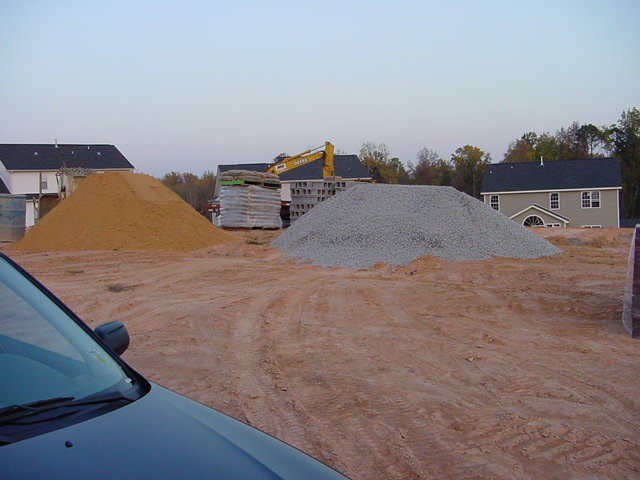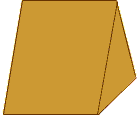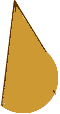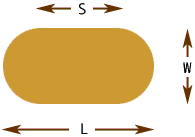I am looking to find a way to measure gravel piles to get yards. They are generaly not square.it would be like pouring sand out of a bag into a pile. they generaly are concave in dimension. can you help. I have attached a picture to show a small scale what I am working on is in much larger volume. Thank you Hi Ron, The best you are going to be able to do is to estimate the number of cubic yards.From the picture you sent any my own experience with sand and gravel piles I think that the base of the pile is approximately a rectangle with semicircles on the ends.If so then the pile can be seen as three pieces. One piece has a rectangular base and is triangular when viewed from the end.The other two pieces are parts of cones.If my approximation seems reasonable to you then you can calculate the volume as follows.As shown in the diagram let W be the width of the pile, L be the length of the pile and S be the elngth of the rectangular part of the base. Let H be the height of the pile. All of these measurements need to be in yards. Let R = W/2 then the volume of the pile in cubic yards is V = 1/2WHS + 1/3π R2H where π is approximately 3.14 If you decide to measure a pile and use this expression let me know what measurements you get. If I am correct in my assumptions then W should be approximately equal to L - S. Harley Go to Math Central